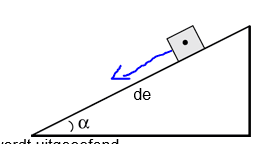# How to calculate the gravity on a hill?

• caspeerrr

#### caspeerrr

Member advised to use the homework template for posts in the homework sections of PF.
The question is about a box with no movement standing on a hill. The hill has an angle of 25 degrees. The box has a mass of 40 kg.
1. Calculte the gravity
This I still get: F= M x A = 40 x 9,81 = 3,9 x 10^2
The next question tough:
2. Calculate the component Fgravity,x off the gravity alongside the hill.
The component I think they ment is marked on the picture included.

What I did was 390 / sin(25) because the opposite is known (the gravity) and you want to calculate the hypotenuse. The answer indicates this as the right answer tough: Fgravity,x = Fgravity sin(a) = 165,8

Why do they multiply when you should divide?
Am I missing something?What I did was 390 / sin(25) because the opposite is known (the gravity) and you want to calculate the hypotenuse.
You want the component of the weight acting down the incline. When finding the component of a vector using a right triangle, the full vector is always the hypotenuse. (The components are always smaller than the full vector.)

You're using the wrong triangle: Draw the weight vector acting straight down. That's the hypotenuse of the correct triangle.

Check this out: Inclined Planes

You want the component of the weight acting down the incline. When finding the component of a vector using a right triangle, the full vector is always the hypotenuse. (The components are always smaller than the full vector.)

You're using the wrong triangle: Draw the weight vector acting straight down. That's the hypotenuse of the correct triangle.

You want the component of the weight acting down the incline. When finding the component of a vector using a right triangle, the full vector is always the hypotenuse. (The components are always smaller than the full vector.)

You're using the wrong triangle: Draw the weight vector acting straight down. That's the hypotenuse of the correct triangle.

Aaah I get it, thank you!

If you find yourself in an exam and can't remember if it's sin or cos... consider what happens if the angle of the slope is reduced towards zero degrees (no slope). The force you calculate down the slope should approach zero. Your calculator will tell you sin(0)=0 but cos(0)=1.